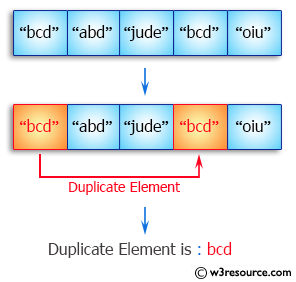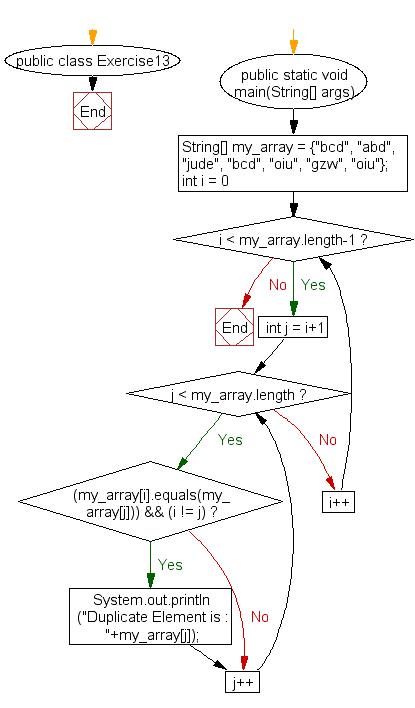﻿ Java exercises: Find the duplicate values of an array of string values - w3resource# Java Array Exercises: Find the duplicate values of an array of string values

## Java Array: Exercise-13 with Solution

Write a Java program to find the duplicate values of an array of string values.

Pictorial Presentation:Sample Solution:

Java Code:

``````public class Exercise13 {
public static void main(String[] args)
{
String[] my_array = {"bcd", "abd", "jude", "bcd", "oiu", "gzw", "oiu"};

for (int i = 0; i < my_array.length-1; i++)
{
for (int j = i+1; j < my_array.length; j++)
{
if( (my_array[i].equals(my_array[j])) && (i != j) )
{
System.out.println("Duplicate Element is : "+my_array[j]);
}
}
}
}
}
```
```

Sample Output:

```Duplicate Element is : bcd
Duplicate Element is : oiu
```

Flowchart:Java Code Editor:

Improve this sample solution and post your code through Disqus

What is the difficulty level of this exercise?

﻿

New Content: Composer: Dependency manager for PHP, R Programming